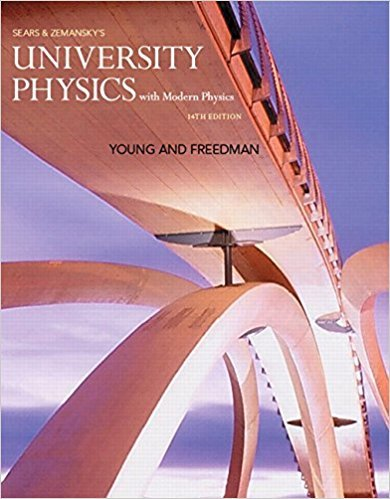# In your physics lab you release a small glider from rest## Problem 2.84 Chapter 2

University Physics with Modern Physics (1) | 14th Edition

• 2901 Step-by-step solutions solved by professors and subject experts
• Get 24/7 help from StudySoup virtual teaching assistantsUniversity Physics with Modern Physics (1) | 14th Edition

4 5 0 247 Reviews
10
0
Problem 2.84

In your physics lab you release a small glider from rest at various points on a long, frictionless air track that is inclined at an angle u above the horizontal. With an electronic photocell, you measure the time t it takes the glider to slide a distance x from the release point to the bottom of the track. Your measurements are given in Fig. P2.84, which shows a second-order polynomial (quadratic) fit to the plotted data. You are asked to find the gliders acceleration, which is assumed to be constant. There is some error in each measurement, so instead of using a single set of x and t values, you can be more accurate if you use graphical methods and obtain your measured value of the acceleration from the graph. (a) How can you re-graph the data so that the data points fall close to a straight line? (Hint: You might want to plot x or t, or both, raised to some power.) (b) Construct the graph you described in part (a) and find the equation for the straight line that is the best fit to the data points. (c) Use the straightline fit from part (b) to calculate the acceleration of the glider. (d) The glider is released at a distance x = 1.35 m from the bottom of the track. Use the acceleration value you obtained in part (c) to calculate the speed of the glider when it reaches the bottom of the track.

Step-by-Step Solution:
Step 1 of 3

● Free Body Diagram: ○ Draw object ○ Include all forces as vectors ○ Resolve into x and y components ○ Draw free body diagram for each object in problem ● Newton's Laws ○ I) Law of inertia: a body at rest, or at motion, remains in motion at a constant velocity unless acted on by an external force ■ Inertial...

Step 2 of 3

Step 3 of 3

##### ISBN: 9780321973610

Unlock Textbook Solution

In your physics lab you release a small glider from rest

×
Get Full Access to University Physics With Modern Physics (1) - 14 Edition - Chapter 2 - Problem 2.84

Get Full Access to University Physics With Modern Physics (1) - 14 Edition - Chapter 2 - Problem 2.84

I don't want to reset my password

Need help? Contact support

Need an Account? Is not associated with an account
We're here to help